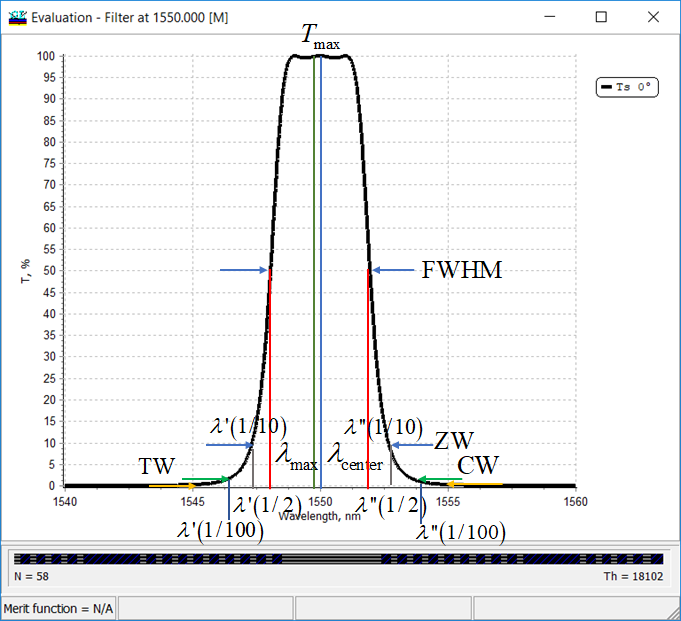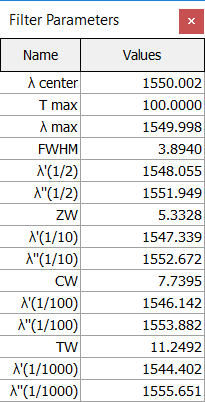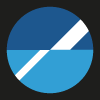### Filter Parameters

 Filter parameters are available through: Evaluation window > Right-click menu > Filter mode This option is mainly aimed at detailed analysis of filter type spectral characteristics but can be used for the analysis of other characteristics as well. The presented data are limited to the first T, R, or BR plots. When activated, it displays in the additional Filter Parameters window such data as central filter wavelength, maximum characteristic value, and wavelength at which this value is achieved, filter widths at various levels, and boundaries of spectral zones at these levels.The main filter parameters are: $$\lambda_{center}$$ - central wavelength, $$T_{max}$$ (or $$R_{max}, BR_{max}$$) - maximum transmittance, reflectance or back side reflectance value, $$\lambda_{max}$$ - wavelength corresponding to the maximum of the spectral characteristic, FWHM - the bandwidth where the filter transmits at least 50% of maximum $$T_{\max}$$, $$\lambda'(1/2)$$ and $$\lambda''(1/2)$$ are boundaries of the FWHM, ZW - the bandwidth where the filter transmits at least 10% of maximum $$T_{\max}$$ (in the case if $$T_{\max}=100\%$$, this is the level $$T=10%$$ (see on the picture above), $$\lambda'(1/10)$$ and $$\lambda''(1/10)$$ are boundaries of the ZW range, CW - the bandwidth where the filter transmits at least 1% of maximum $$T_{\max}$$ (in the case if $$T_{\max}=100\%$$, this is the level $$T=1%$$ (see on the picture above), $$\lambda'(1/100)$$ and $$\lambda''(1/100)$$ are boundaries of the CW range, TW - the bandwidth where the filter transmits at least 0.1% of maximum $$T_{\max}$$ (in the case if $$T_{\max}=100\%$$, this is the level $$T=0.1%$$ (see on the picture above), $$\lambda'(1/1000)$$ and $$\lambda''(1/1000)$$ are boundaries of the TW range.

### Easy to startOptiLayer provides user-friendly interface and a variety of examples allowing even a beginner to effectively start to design and characterize optical coatings.        Read more...

### Docs / SupportComprehensive manual in PDF format and e-mail support help you at each step of your work with OptiLayer.If you are already an experienced user, OptiLayer gives your almost unlimited opportunities in solving all problems arising in design-production chain. Visit our publications page and challenge page.